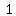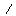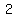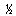# U+00BD VULGAR FRACTION ONE HALF

U+00BD was added to Unicode in version 1.1 (1993). It belongs to the blockLatin-1 Supplement in the Basic Multilingual Plane.

This character is a Other Number and is commonly used, that is, in no specific script. The codepoint has the Numeric value 1/2.

One half is the irreducible fraction resulting from dividing one by two (½), or any number by its double; multiplication by one half is equivalent to division by two. Conversely, division by one half is equivalent to multiplication by two, or "doubling". One half appears often in mathematical equations, recipes, measurements, etc. Half can also be said to be one part of something divided into two equal parts.

For instance, the area S of a triangle is computed

S = ½ × base × perpendicular height.

One half also figures in the formula for calculating figurate numbers, such as triangular numbers and pentagonal numbers:

½ × n [(s - 2) n - (4 - s)]

and in the formula for computing magic constants for magic squares

M2(n) = ½ × [n (n2 + 1 )].

The Riemann hypothesis states that every nontrivial complex root of the Riemann zeta function has a real part equal to ½.

One half has two different decimal expansions, the familiar 0.5 and the recurring 0.49999999... It has a similar pair of expansions in any even base. It is a common trap to believe these expressions represent distinct numbers: see the proof that 0.999... equals 1 for detailed discussion of a related case.

## Representations

System Representation
189
UTF-8 C2 BD
UTF-16 00 BD
UTF-32 00 00 00 BD
URL-Quoted %C2%BD
HTML-Escape &#x00BD;
Wrong windows-1252 Mojibake Â½
HTML-Escape &frac12;
HTML-Escape &half;
digraph 12
LaTeX \textonehalf
Encoding: EUC-KR (hex bytes) A8 F6
Encoding: ISO-8859-13 (hex bytes) BD
Encoding: ISO-8859-3 (hex bytes) BD
Encoding: ISO-8859-7 (hex bytes) BD
Encoding: ISO-8859-8 (hex bytes) BD
Encoding: WINDOWS-1252 (hex bytes) BD
Encoding: WINDOWS-1253 (hex bytes) BD
Encoding: WINDOWS-1254 (hex bytes) BD
Encoding: WINDOWS-1255 (hex bytes) BD
Encoding: WINDOWS-1256 (hex bytes) BD
Encoding: WINDOWS-1257 (hex bytes) BD
Encoding: WINDOWS-1258 (hex bytes) BD

## Complete Record

Property Value
Age (age) 1.1
Unicode Name (na) VULGAR FRACTION ONE HALF
Unicode 1 Name (na1) FRACTION ONE HALF
Block (blk) Latin_1_Sup
General Category (gc) Other Number
Script (sc) Common
Bidirectional Category (bc) Other Neutral
Combining Class (ccc) Not Reordered
Decomposition Type (dt) Fraction
Decomposition Mapping (dm)Lowercase (Lower)
Simple Lowercase Mapping (slc)Lowercase Mapping (lc)Uppercase (Upper)
Simple Uppercase Mapping (suc)Uppercase Mapping (uc)Simple Titlecase Mapping (stc)Titlecase Mapping (tc)Case Folding (cf)ASCII Hex Digit (AHex)
Alphabetic (Alpha)
Bidi Control (Bidi_C)
Bidi Mirrored (Bidi_M)
Bidi Paired Bracket (bpb)Bidi Paired Bracket Type (bpt) None
Cased (Cased)
Composition Exclusion (CE)
Case Ignorable (CI)
Full Composition Exclusion (Comp_Ex)
Changes When Casefolded (CWCF)
Changes When Casemapped (CWCM)
Changes When NFKC Casefolded (CWKCF)
Changes When Lowercased (CWL)
Changes When Titlecased (CWT)
Changes When Uppercased (CWU)
Dash (Dash)
Deprecated (Dep)
Default Ignorable Code Point (DI)
Diacritic (Dia)
East Asian Width (ea) Ambiguous
Extender (Ext)
FC NFKC Closure (FC_NFKC)Grapheme Cluster Break (GCB) Any
Grapheme Base (Gr_Base)
Grapheme Extend (Gr_Ext)
Hex Digit (Hex)
Hangul Syllable Type (hst) Not Applicable
Hyphen (Hyphen)
ID Continue (IDC)
Ideographic (Ideo)
ID Start (IDS)
IDS Binary Operator (IDSB)
IDS Trinary Operator and (IDST)
InMC (InMC)
Indic Positional Category (InPC) NA
Indic Syllabic Category (InSC) Other
ISO 10646 Comment (isc)
Joining Group (jg) No_Joining_Group
Join Control (Join_C)
Jamo Short Name (JSN)
Joining Type (jt) Non Joining
Line Break (lb) Ambiguous
Logical Order Exception (LOE)
Math (Math)
Noncharacter Code Point (NChar)
NFC Quick Check (NFC_QC) Yes
NFD Quick Check (NFD_QC) Yes
NFKC Casefold (NFKC_CF)NFKC Quick Check (NFKC_QC) No
NFKD Quick Check (NFKD_QC) No
Numeric Type (nt) Numeric
Numeric Value (nv) 1/2
Other Alphabetic (OAlpha)
Other Default Ignorable Code Point (ODI)
Other Grapheme Extend (OGr_Ext)
Other ID Continue (OIDC)
Other ID Start (OIDS)
Other Lowercase (OLower)
Other Math (OMath)
Other Uppercase (OUpper)
Pattern Syntax (Pat_Syn)
Pattern White Space (Pat_WS)
Quotation Mark (QMark)
Sentence Break (SB) Other
Simple Case Folding (scf)Script Extension (scx) Common
Soft Dotted (SD)
STerm (STerm)
Terminal Punctuation (Term)
Unified Ideograph (UIdeo)
Variation Selector (VS)
Word Break (WB) Other
White Space (WSpace)
XID Continue (XIDC)
XID Start (XIDS)
Expands On NFC (XO_NFC)
Expands On NFD (XO_NFD)
Expands On NFKC (XO_NFKC)
Expands On NFKD (XO_NFKD)